Research

## FermiNet: Quantum Physics and Chemistry from First Principles

October 19, 2020Research

## FermiNet: Quantum Physics and Chemistry from First Principles

October 19, 2020

In an article recently published in Physical Review Research, we show how deep learning can help solve the fundamental equations of quantum mechanics for real-world systems. Not only is this an important fundamental scientific question, but it also could lead to practical uses in the future, allowing researchers to prototype new materials and chemical syntheses in silico before trying to make them in the lab. Today we are also releasing the code from this study so that the computational physics and chemistry communities can build on our work and apply it to a wide range of problems. We’ve developed a new neural network architecture, the Fermionic Neural Network or FermiNet, which is well-suited to modeling the quantum state of large collections of electrons, the fundamental building blocks of chemical bonds. The FermiNet was the first demonstration of deep learning for computing the energy of atoms and molecules from first principles that was accurate enough to be useful, and it remains the most accurate neural network method to date. We hope the tools and ideas developed in our AI research at DeepMind can help solve fundamental problems in the natural sciences, and the FermiNet joins our work on protein folding, glassy dynamics, lattice quantum chromodynamics and many other projects in bringing that vision to life.

#### A Brief History of Quantum Mechanics

Mention “quantum mechanics” and you are more likely to inspire confusion than anything else. The phrase conjures up images of Schrödinger’s cat, which can paradoxically be both alive and dead, and fundamental particles that are also, somehow, waves.  In quantum systems, a particle such as an electron doesn’t have an exact location, as it would in a classical description. Instead, its position is described by a probability cloud - it’s smeared out in all places it’s allowed to be. This counterintuitive state of affairs led Richard Feynman to declare: “If you think you understand quantum mechanics, you don’t understand quantum mechanics.” Despite this spooky weirdness, the meat of the theory can be reduced down to just a few straightforward equations. The most famous of these, the Schrödinger equation, describes the behavior of particles at the quantum scale in the same way that Newton’s laws describe the behavior of objects at our more familiar human scale. While the interpretation of this equation can cause endless head-scratching, the math is much easier to work with, leading to the common exhortation from professors to “shut up and calculate” when pressed with thorny philosophical questions from students.

These equations are sufficient to describe the behavior of all the familiar matter we see around us at the level of atoms and nuclei. Their counterintuitive nature leads to all sorts of exotic phenomena: superconductors, superfluids, lasers and semiconductors are only possible because of quantum effects. But even the humble covalent bond - the basic building block of chemistry - is a consequence of the quantum interactions of electrons. Once these rules were worked out in the 1920s, scientists realised that, for the first time, they had a detailed theory of how chemistry works. In principle, they could just set up these equations for different molecules, solve for the energy of the system, and figure out which molecules were stable and which reactions would happen spontaneously. But when they sat down to actually calculate the solutions to these equations, they found that they could do it exactly for the simplest atom (hydrogen) and virtually nothing else. Everything else was too complicated.

The heady optimism of those days was nicely summed up by Paul Dirac:

The underlying physical laws necessary for the mathematical theory of a large part of physics and the whole of chemistry are thus completely known, and the difficulty is only that the exact application of these laws leads to equations much too complicated to be soluble. It therefore becomes desirable that approximate practical methods of applying quantum mechanics should be developed
Paul Dirac, 1929

Many took up Dirac’s charge, and soon physicists built mathematical techniques that could approximate the qualitative behavior of molecular bonds and other chemical phenomena. These methods started from an approximate description of how electrons behave that may be familiar from introductory chemistry. In this description, each electron is assigned to a particular orbital, which gives the probability of a single electron being found at any point near an atomic nucleus. The shape of each orbital then depends on the average shape of all other orbitals. As this “mean field” description treats each electron as being assigned to just one orbital, it is a very incomplete picture of how electrons actually behave. Nevertheless, it is enough to estimate the total energy of a molecule with only about 0.5% error.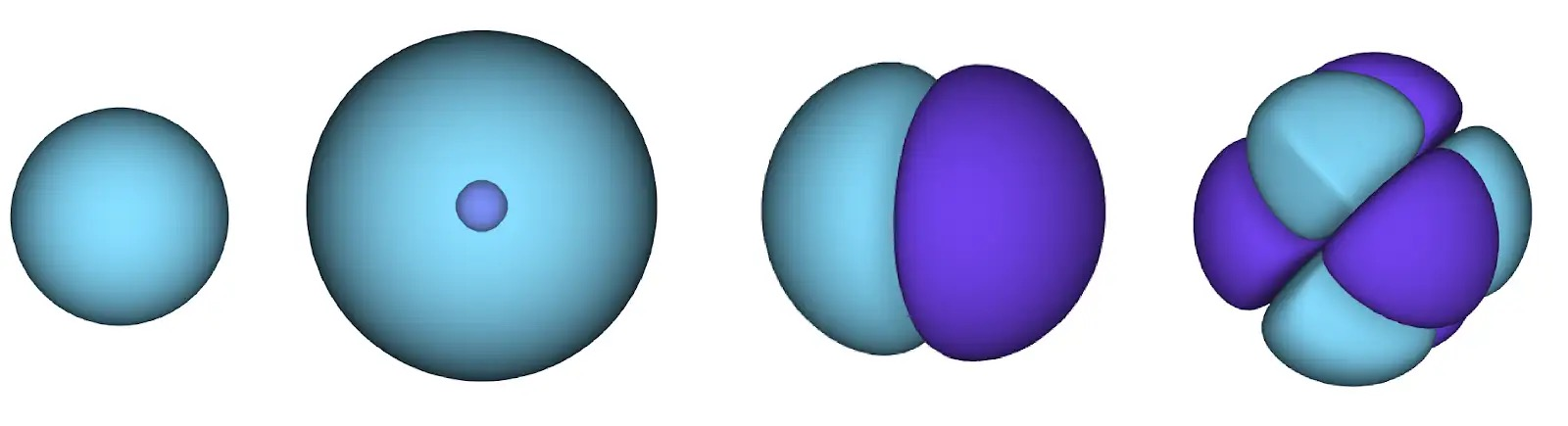Figure 1 - Atomic orbitals. The surface denotes the area of high probability of finding an electron. In the blue region the wavefunction is positive, while in the purple region it is negative.

Unfortunately, 0.5% error still isn’t enough to be useful to the working chemist. The energy in molecular bonds is just a tiny fraction of the total energy of a system, and correctly predicting whether a molecule is stable can often depend on just 0.001% of the total energy of a system, or about 0.2% of the remaining “correlation” energy. For instance, while the total energy of the electrons in a butadiene molecule is almost 100,000 kilocalories per mole, the difference in energy between different possible shapes of the molecule is just 1 kilocalorie per mole. That means that if you want to correctly predict butadiene’s natural shape, then the same level of precision is needed as measuring the width of a football field down to the millimeter.

With the advent of digital computing after World War II, scientists developed a whole menagerie of computational methods that went beyond this mean field description of electrons. While these methods come in a bewildering alphabet soup of abbreviations, they all generally fall somewhere on an axis that trades off accuracy with efficiency. At one extreme, there are methods that are essentially exact, but scale worse than exponentially with the number of electrons, making them impractical for all but the smallest molecules. At the other extreme are methods that scale linearly, but are not very accurate. These computational methods have had an enormous impact on the practice of chemistry - the 1998 Nobel Prize in chemistry was awarded to the originators of many of these algorithms.

#### Fermionic Neural Networks

Despite the breadth of existing computational quantum mechanical tools, we felt a new method was needed to address the problem of efficient representation. There’s a reason that the largest quantum chemical calculations only run into the tens of thousands of electrons for even the most approximate methods, while classical chemical calculation techniques like molecular dynamics can handle millions of atoms. The state of a classical system can be described easily - we just have to track the position and momentum of each particle. Representing the state of a quantum system is far more challenging. A probability has to be assigned to every possible configuration of electron positions. This is encoded in the wavefunction, which assigns a positive or negative number to every configuration of electrons, and the wavefunction squared gives the probability of finding the system in that configuration. The space of all possible configurations is enormous - if you tried to represent it as a grid with 100 points along each dimension, then the number of possible electron configurations for the silicon atom would be larger than the number of atoms in the universe!

This is exactly where we thought deep neural networks could help. In the last several years, there have been huge advances in representing complex, high-dimensional probability distributions with neural networks. We now know how to train these networks efficiently and scalably. We surmised that, given these networks have already proven their mettle at fitting high-dimensional functions in artificial intelligence problems, maybe they could be used to represent quantum wavefunctions as well. We were not the first people to think of this - researchers such as Giuseppe Carleo and Matthias Troyer and others have shown how modern deep learning could be used for solving idealised quantum problems. We wanted to use deep neural networks to tackle more realistic problems in chemistry and condensed matter physics, and that meant including electrons in our calculations.

There is just one wrinkle when dealing with electrons. Electrons must obey the Pauli exclusion principle, which means that they can’t be in the same space at the same time. This is because electrons are a type of particle known as fermions, which include the building blocks of most matter - protons, neutrons, quarks, neutrinos, etc. Their wavefunction must be antisymmetric - if you swap the position of two electrons, the wavefunction gets multiplied by -1. That means that if two electrons are on top of each other, the wavefunction (and the probability of that configuration) will be zero.

This meant we had to develop a new type of neural network that was antisymmetric with respect to its inputs, which we have dubbed the Fermionic Neural Network, or FermiNet. In most quantum chemistry methods, antisymmetry is introduced using a function called the determinant. The determinant of a matrix has the property that if you swap two rows, the output gets multiplied by -1, just like a wavefunction for fermions. So you can take a bunch of single-electron functions, evaluate them for every electron in your system, and pack all of the results into one matrix. The determinant of that matrix is then a properly antisymmetric wavefunction. The major limitation of this approach is that the resulting function - known as a Slater determinant - is not very general. Wavefunctions of real systems are usually far more complicated. The typical way to improve on this is to take a large linear combination of Slater determinants - sometimes millions or more - and add some simple corrections based on pairs of electrons. Even then, this may not be enough to accurately compute energies.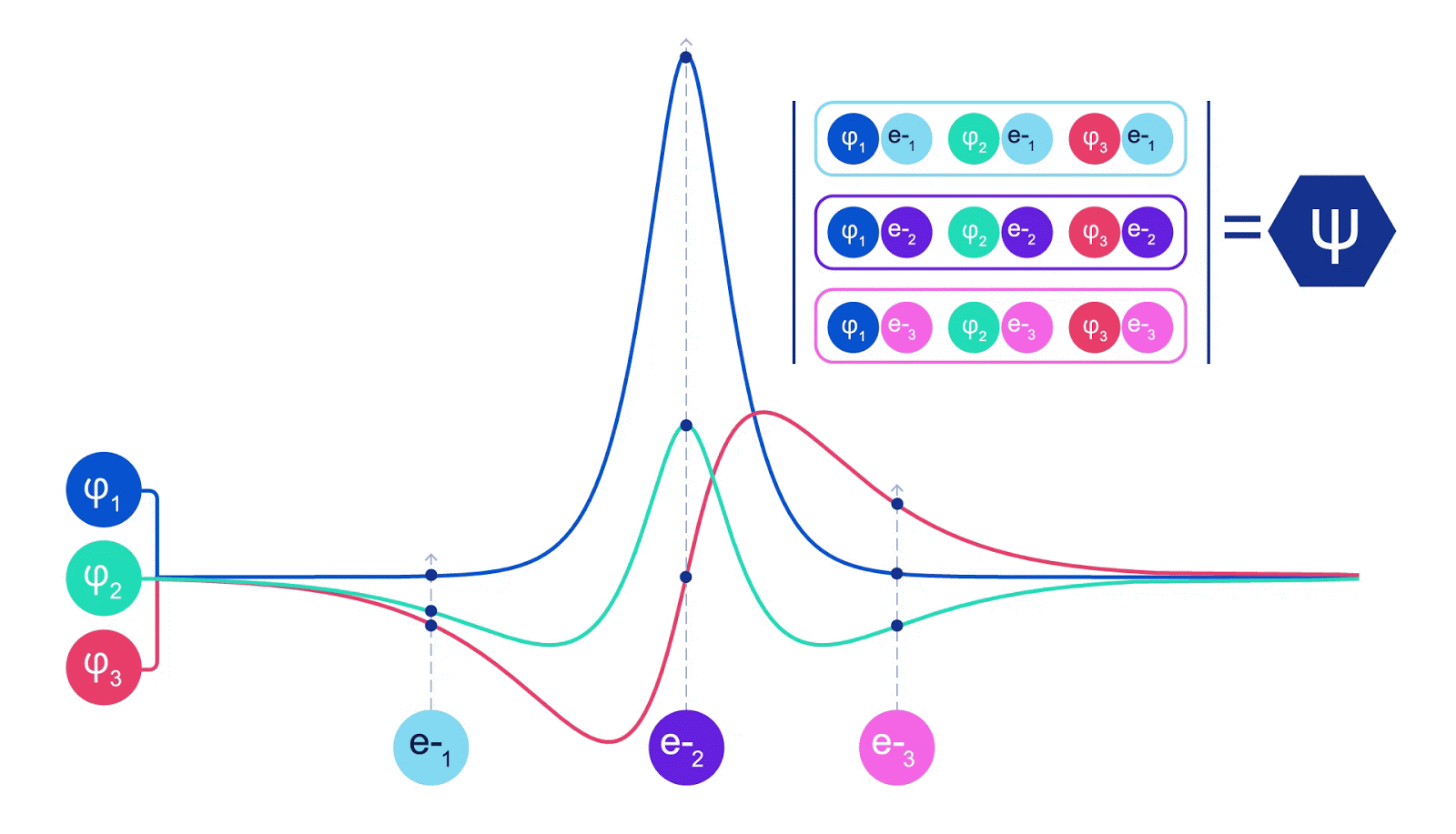Figure 2 - Illustration of a Slater determinant. Each curve is a slice through one of the orbitals from Figure 1. When electrons 1 and 2 swap positions, the rows of the Slater determinant swap, and the wavefunction is multiplied by -1. This guarantees that the Pauli exclusion principle is obeyed.

Deep neural networks can often be far more efficient at representing complex functions than linear combinations of basis functions. In the FermiNet, this is achieved by making each function going into the determinant a function of all electrons (1). This goes far beyond methods that just use one- and two-electron functions. The FermiNet has a separate stream of information for each electron. Without any interaction between these streams, the network would be no more expressive than a conventional Slater determinant. To go beyond this, we average together information from across all streams at each layer of the network, and pass this information to each stream at the next layer. That way, these streams have the right symmetry properties to create an antisymmetric function. This is similar to how graph neural networks aggregate information at each layer. Unlike the Slater determinants, FermiNets are universal function approximators, at least in the limit where the neural network layers become wide enough. That means that, if we can train these networks correctly, they should be able to fit the nearly-exact solution to the Schrödinger equation.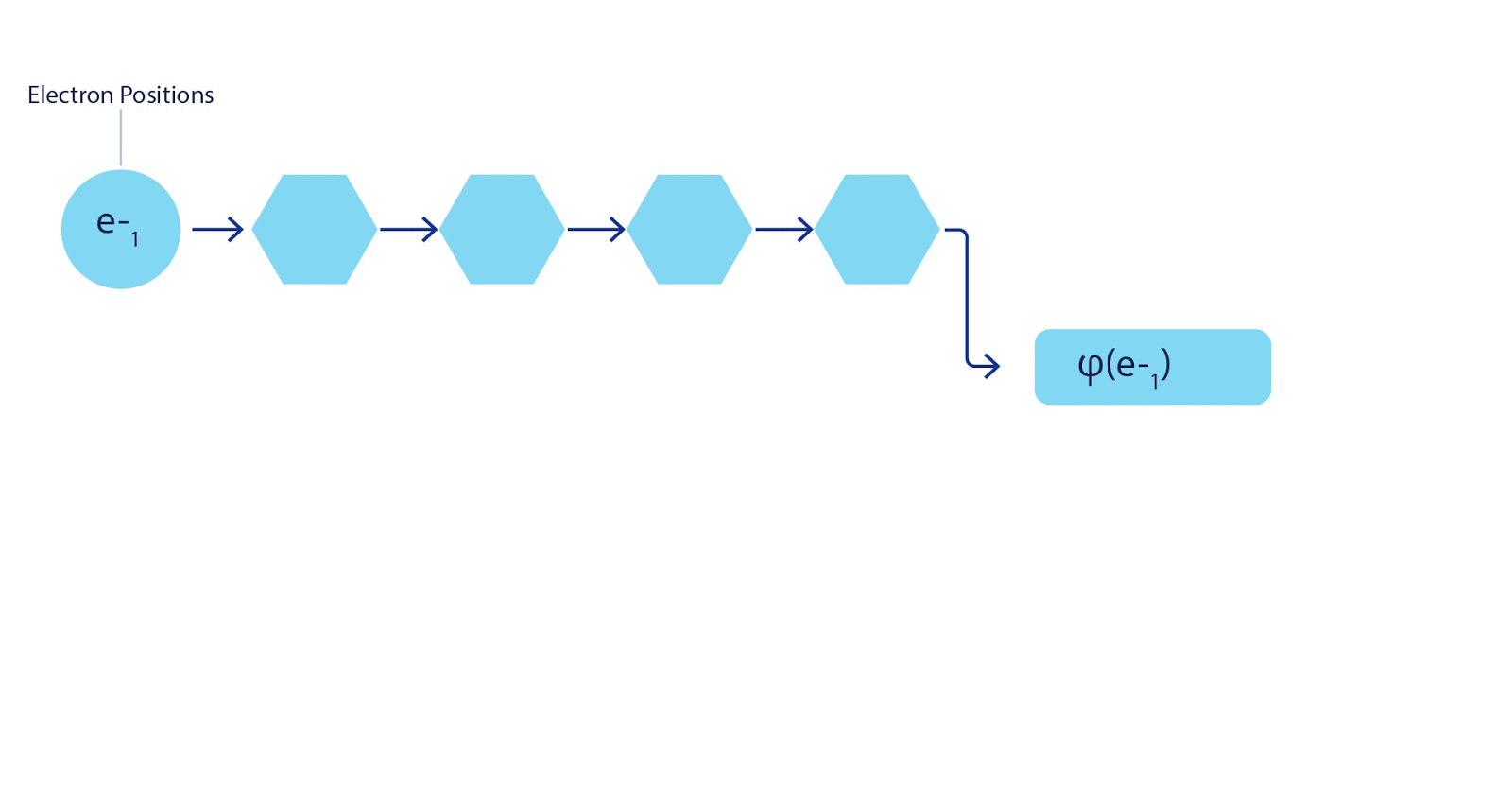Fig 3 - Illustration of the FermiNet. A single stream of the network (blue, purple or pink) functions very similarly to a conventional orbital. The FermiNet introduces symmetric interactions between streams, making the wavefunction far more general and expressive. Just like a conventional Slater determinant, swapping two electron positions still leads to swapping two rows in the determinant, and multiplying the overall wavefunction by -1.

We fit the FermiNet by minimising the energy of the system. To do that exactly, we would need to evaluate the wavefunction at all possible configurations of electrons, so we have to do it approximately instead. We pick a random selection of electron configurations, evaluate the energy locally at each arrangement of electrons, add up the contributions from each arrangement and minimise this instead of the true energy. This is known as a Monte Carlo method, because it’s a bit like a gambler rolling dice over and over again. While it is approximate, if we need to make it more accurate we can always roll the dice again. Since the wavefunction squared gives the probability of observing an arrangement of particles in any location, it is most convenient to generate samples from the wavefunction itself - essentially, simulating the act of observing the particles. While most neural networks are trained from some external data, in our case the inputs used to train the neural network are generated by the neural network itself. It’s a bit like pulling yourself up by your own bootstraps, and it means that we don’t need any training data other than the positions of the atomic nuclei that the electrons are dancing around. The basic idea, known as variational quantum Monte Carlo (or VMC for short), has been around since the ‘60s, and it is generally considered a cheap but not very accurate way of computing the energy of a system. By replacing the simple wavefunctions based on Slater determinants with the FermiNet, we have dramatically increased the accuracy of this approach on every system we’ve looked at.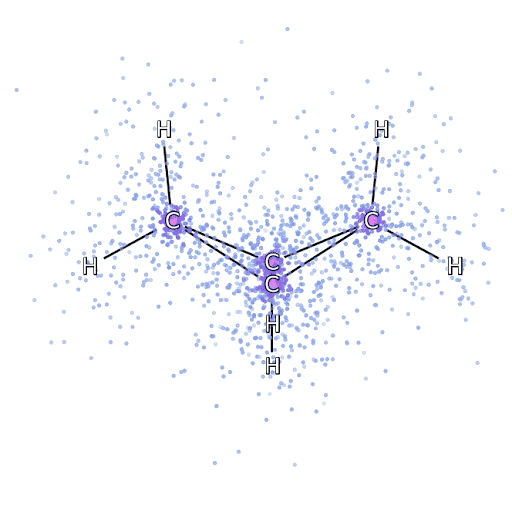Fig 4 - Simulated electrons sampled from the FermiNet move around the bicyclobutane molecule.

To make sure that the FermiNet really does represent an advance in the state of the art, we started by investigating simple, well-studied systems, like atoms in the first row of the periodic table (hydrogen through neon). These are small systems - 10 electrons or fewer - and simple enough that they can be treated by the most accurate (but exponential scaling) methods. The FermiNet outperforms comparable VMC calculations by a wide margin - often cutting the error relative to the exponentially-scaling calculations by half or more. On larger systems, the exponentially-scaling methods become intractable, so instead we use the “coupled cluster” method as a baseline. This method works well on molecules in their stable configuration, but struggles when bonds get stretched or broken, which is critical for understanding chemical reactions. While it scales much better than exponentially, the particular coupled cluster method we used still scales as the number of electrons raised to the seventh power, so it can only be used for medium-sized molecules. We applied the FermiNet to progressively larger molecules, starting with lithium hydride and working our way up to bicyclobutane, the largest system we looked at, with 30 electrons. On the smallest molecules, the FermiNet captured an astounding 99.8% of the difference between the coupled cluster energy and the energy you get from a single Slater determinant. On bicyclobutane, the FermiNet still captured 97% or more of this correlation energy - a huge accomplishment for a supposedly “cheap but inaccurate” approach.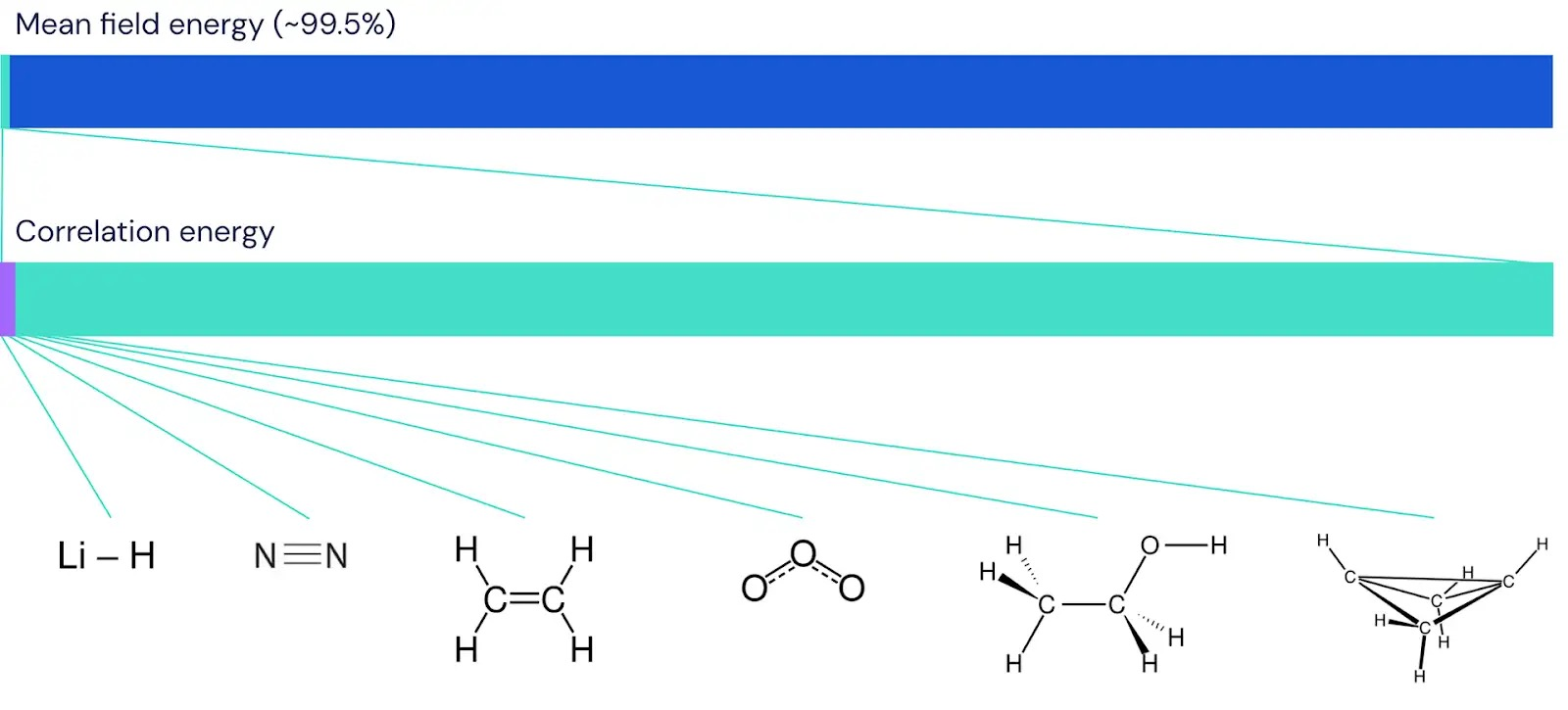Fig 5 - Graphic depiction of the fraction of correlation energy that the FermiNet captures on molecules. The purple bar indicates 99% of correlation energy. Left to right: lithium hydride, nitrogen, ethene, ozone, ethanol and bicyclobutane.

While coupled cluster methods work well for stable molecules, the real frontier in computational chemistry is in understanding how molecules stretch, twist and break. There, coupled cluster methods often struggle, so we have to compare against as many baselines as possible to make sure we get a consistent answer. We looked at two benchmark stretched systems - the nitrogen molecule (N2) and the hydrogen chain with 10 atoms, (H10). Nitrogen is an especially challenging molecular bond, because each nitrogen atom contributes 3 electrons. The hydrogen chain, meanwhile, is of interest for understanding how electrons behave in materials, for instance predicting whether or not a material will conduct electricity. On both systems, coupled cluster did well at equilibrium, but had problems as the bonds were stretched. Conventional VMC calculations did poorly across the board. But the FermiNet was among the best methods investigated, no matter the bond length.

#### Conclusions

We think the FermiNet is the start of great things to come for the fusion of deep learning and computational quantum chemistry. Most of the systems we’ve looked at so far are well-studied and well-understood. But just as the first good results with deep learning in other fields led to a burst of follow-up work and rapid progress, we hope that the FermiNet will inspire lots of work on scaling up and many ideas for new, even better network architectures. Already, since we first put our work on arXiv last year, other groups have shared their approaches to applying deep learning to first-principles calculations on the many-electron problem. We have also just scratched the surface of computational quantum physics, and look forward to applying the FermiNet to tough problems in material science and condensed matter physics as well. Mostly, we hope that by releasing the source code used in our experiments, we can inspire other researchers to build on our work and try out new applications we haven’t even dreamed of.

No items found.